# 二分图（三）——KM算法

KM算法：求在一个二分图的完备匹配中的最大权值匹配的算法。（下文简称为最佳完备匹配）

1.运用贪心算法初始化标杆。
2.运用匈牙利算法找到完备匹配。
3.如果找不到，则通过修改标杆，增加一些边。
4.重复2，3的步骤，找到完备匹配时可结束。

x1 x2 x3 x4 x5
y1 7 3 14 19 23
y2 8 10 15 18 20
y3 6 8 12 16 19
y4 4 9 13 20 25
y5 2 7 12 10 15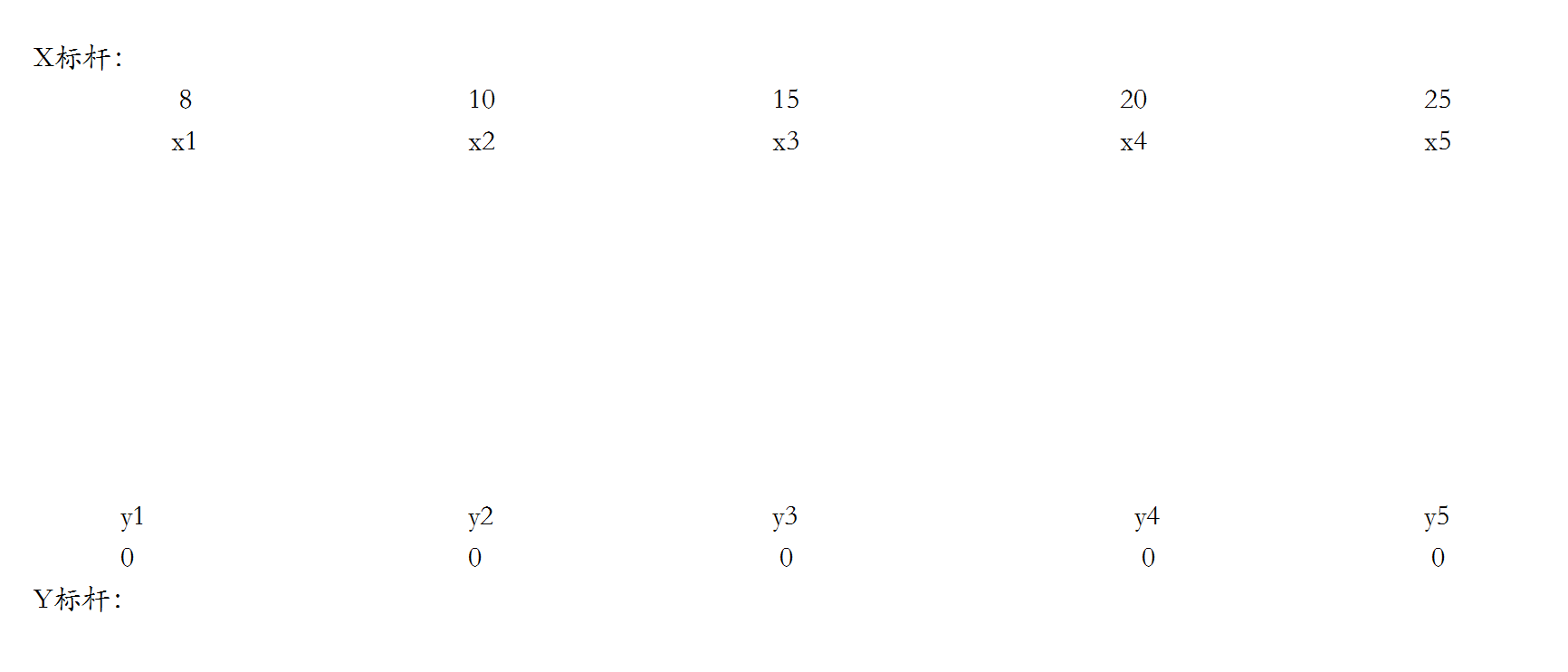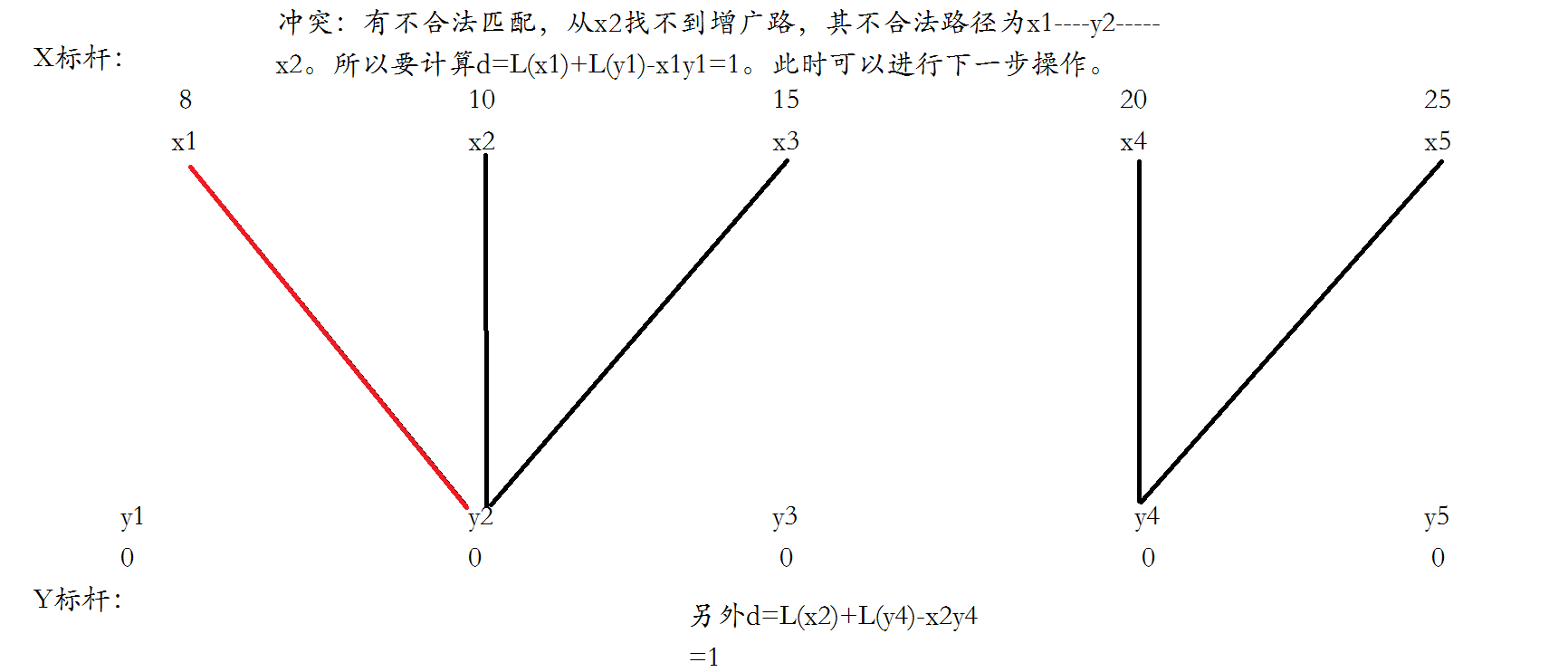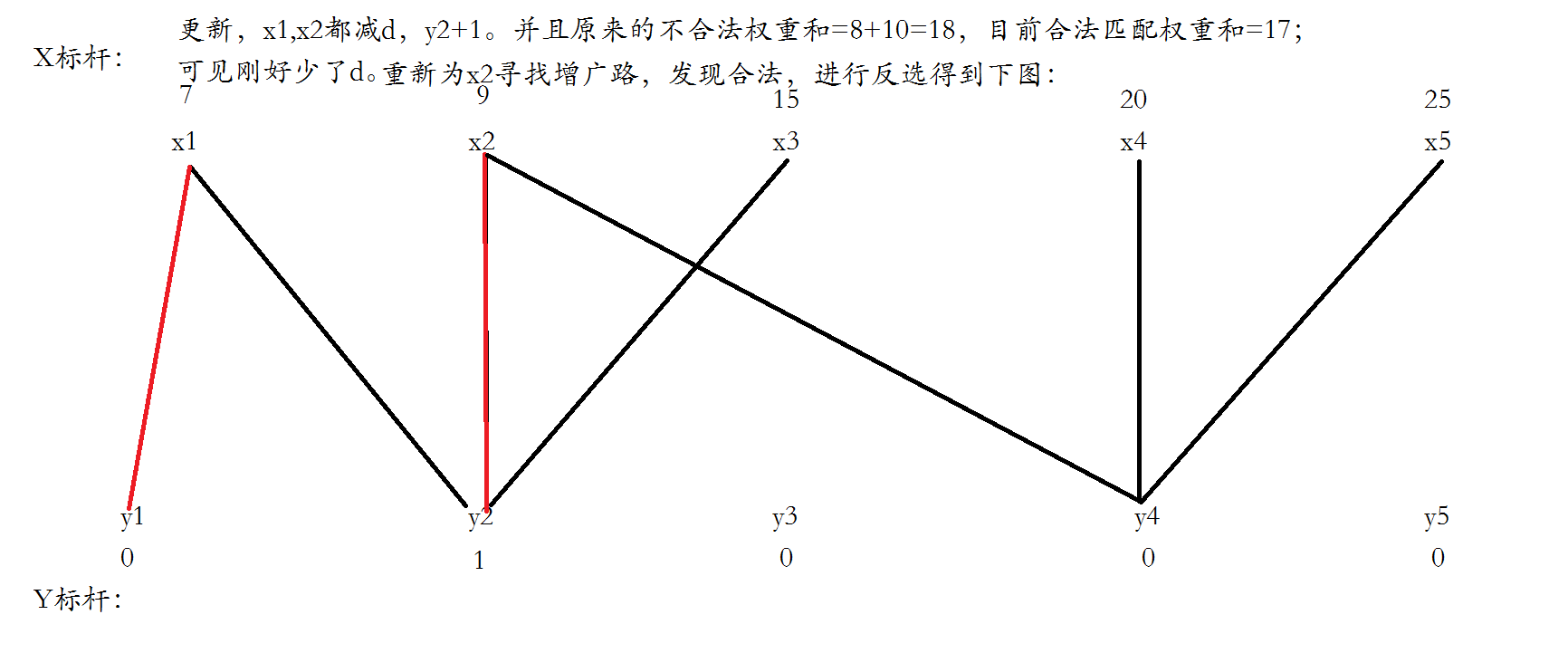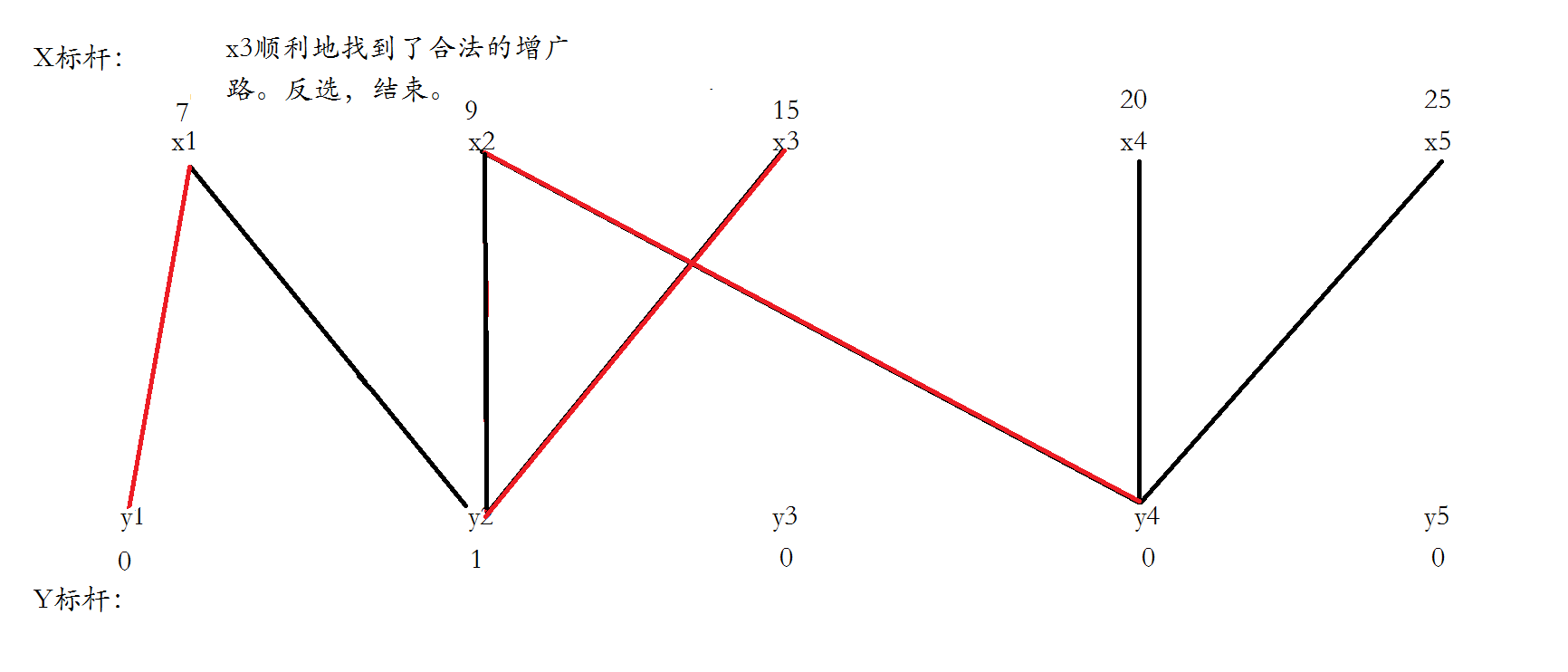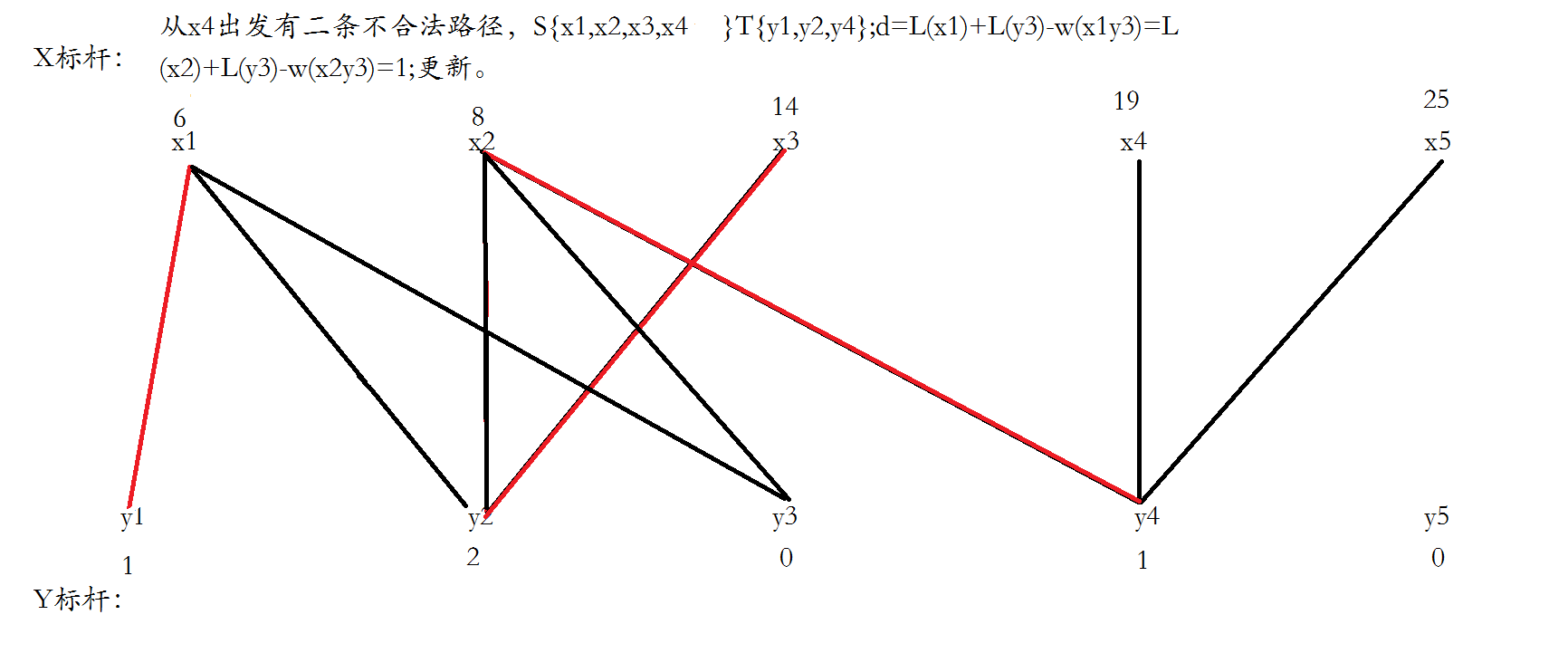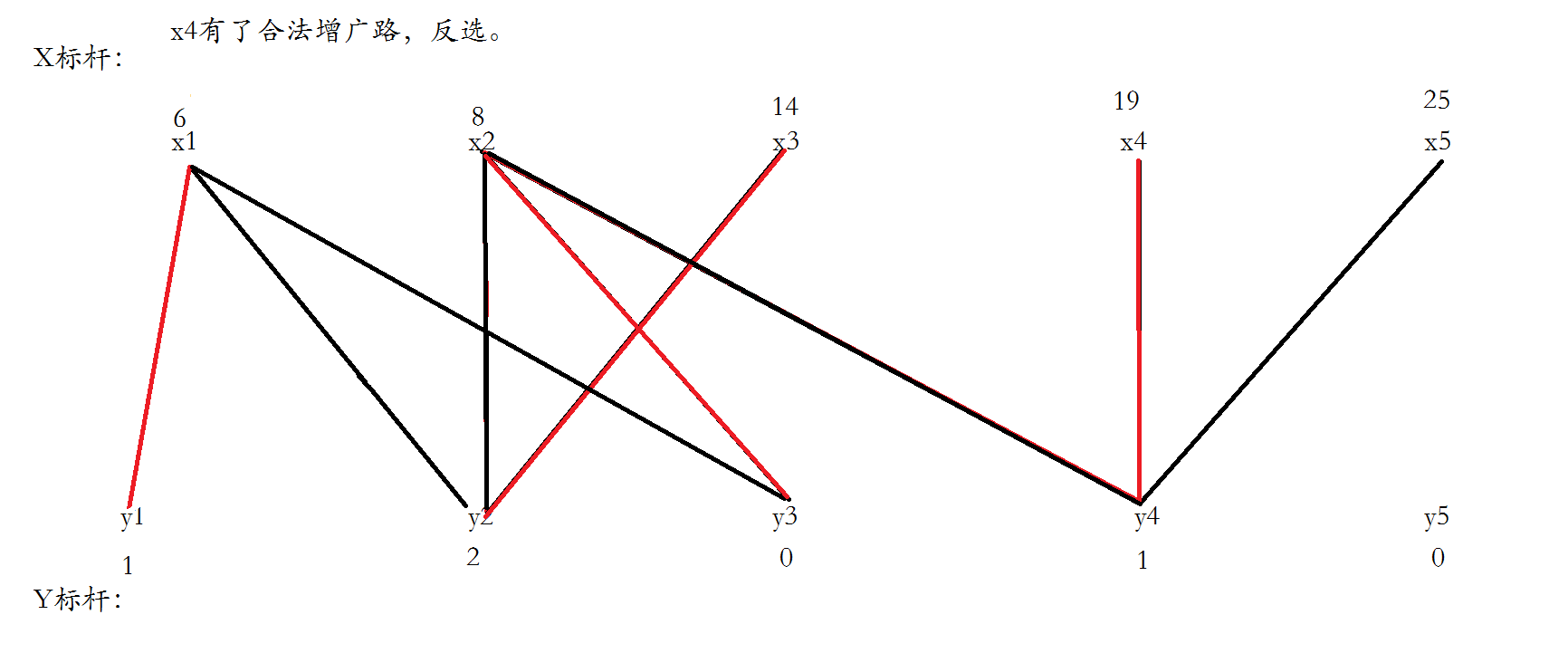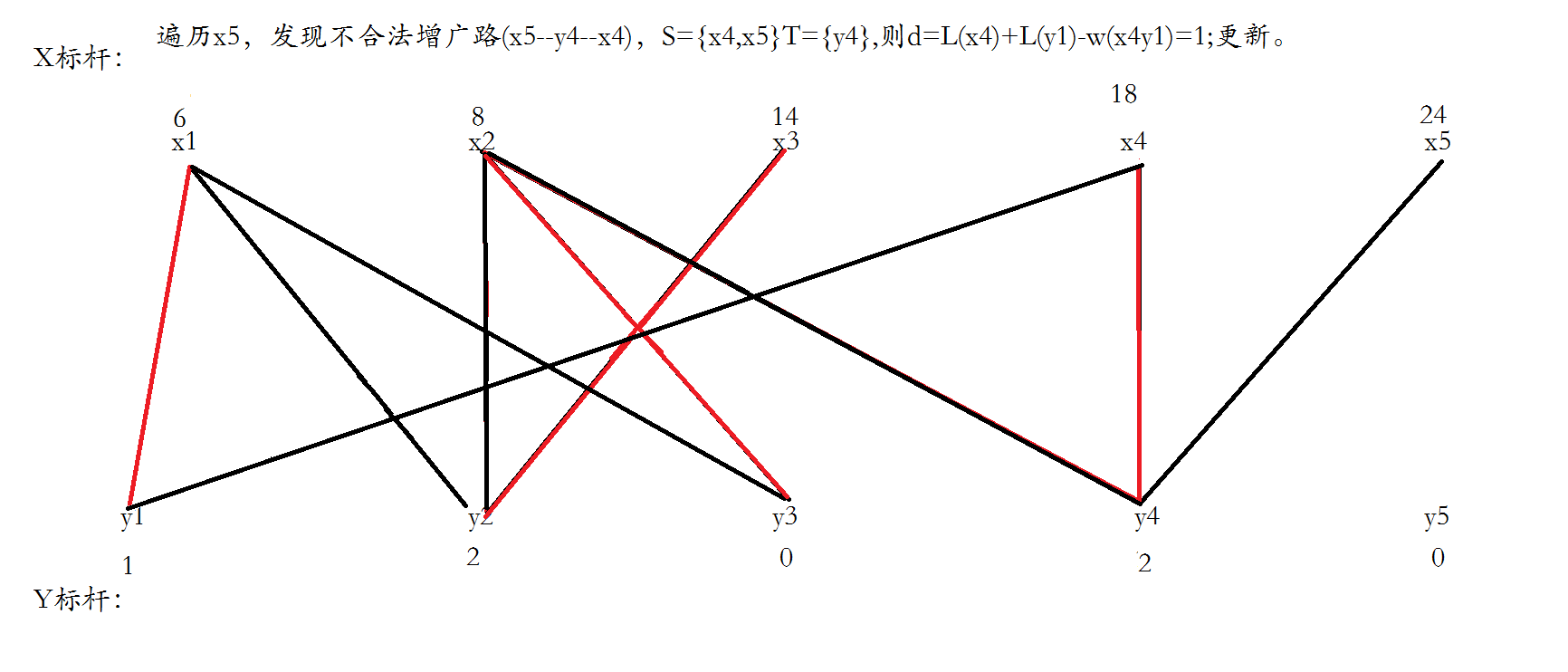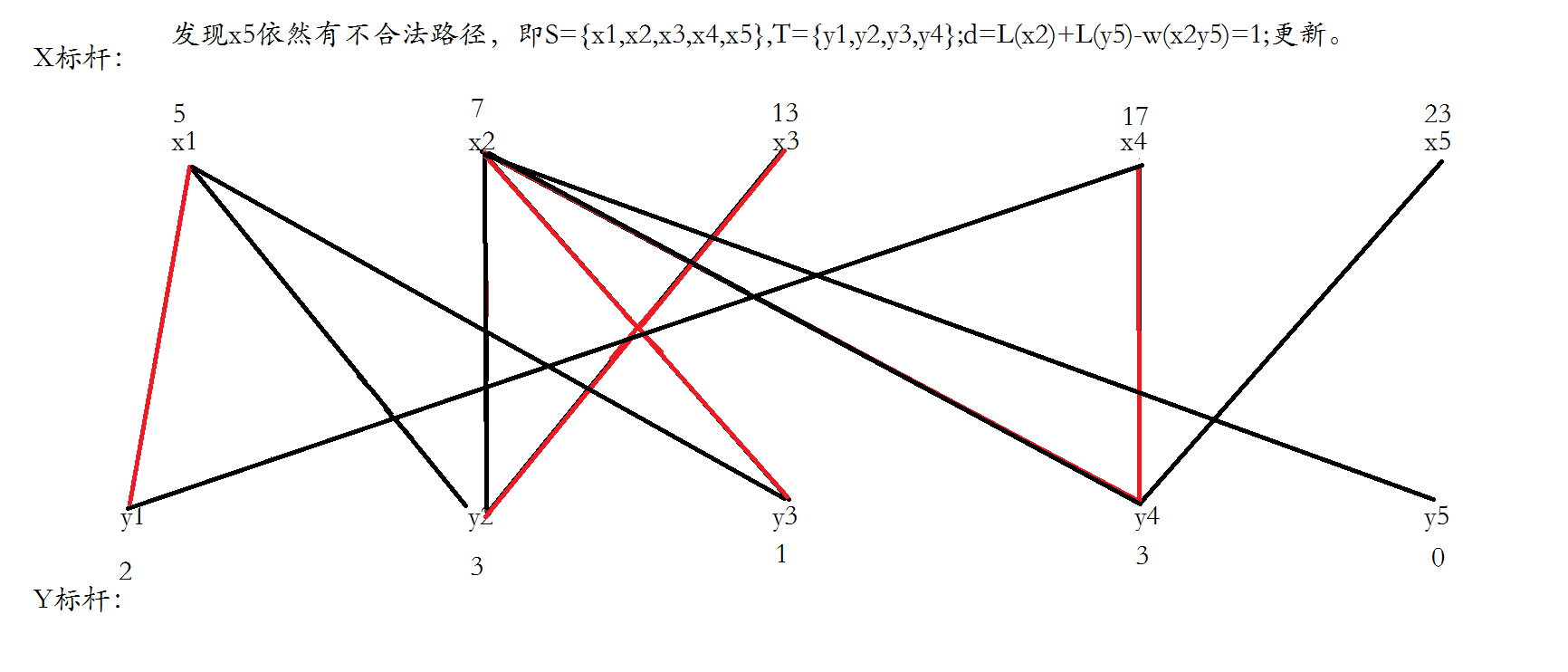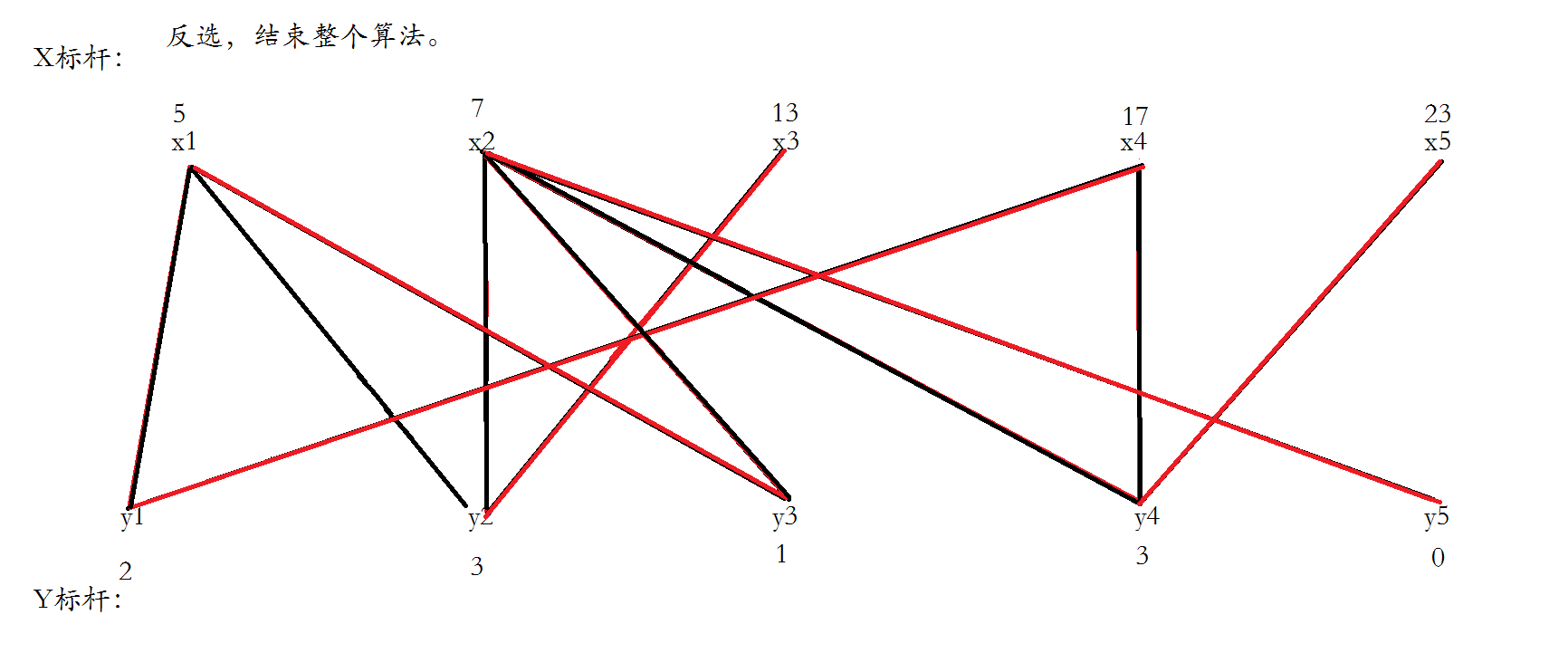#include <iostream>
#include <ctime>
#include <algorithm>
#include <cmath>
#include <cstdio>
#include <cstring>
#include <cstdlib>
#include <queue>
#include <iomanip>
#include <stack>
using namespace std;
int w;
const int inf=(1<<20)-1;
int m,n;//n左m右
int cx,cy;//顶标
bool usex,usey;//本回合使用的x，y
bool dfs(int u){
usex[u]=1;
for(int i=1;i<=m;i++)
if(!usey[i]&&cx[u]+cy[i]==w[u][i]){
usey[i]=1;
return 1;
}
}
return 0;
}
int KM(){
memset(cy,0,sizeof(cy));
memset(cx,-1,sizeof(cx));
for(int i=1;i<=n;i++)
for(int j=1;j<=m;j++)
cx[i]=max(cx[i],w[i][j]);
for(int i=1;i<=n;i++){
while(1){
int d=inf;
memset(usex,0,sizeof(usex));
memset(usey,0,sizeof(usey));
if(dfs(i))break;
for(int i=1;i<=n;i++)
if(usex[i])
for(int j=1;j<=m;j++)
if(!usey[j])d=min(d,cx[i]+cy[j]-w[i][j]);
if(d==inf)return -1;
for(int i=1;i<=n;i++)
if(usex[i])cx[i]-=d;
for(int i=1;i<=m;i++)
if(usey[i])cy[i]+=d;
}
}
int ans=0;
for(int i=1;i<=m;i++){
}
return ans;
}
int main (){
while(~scanf("%d%d",&n,&m)){
memset(w,0,sizeof(w));
int a,b,c;
for(int i=1;i<=n;i++)
for(int j=1;j<=m;j++)
scanf("%d",&w[i][j]);
printf("%d\n",KM());
}
return 0;
}


06-013508

#### KM算法详解+模板12-26655

#### 我对KM算法的理解

08-292329

#### 二分图最大权匹配 KM算法

07-1420

#### Kotlin 入门第一天：函数实现的几种方式

05-201万+

#### 二部图（二分图）总结

08-081万+

#### 二分图的定义和判定

01-304万+

#### 如何实现按距离排序、范围查找

07-1811万+

#### 趣写算法系列之--匈牙利算法

07-101010

#### 6年拉力工作经验，学了阿里P8级架构师的7+1+1落地项目，跳槽阿里年薪直接40W+©️2020 CSDN 皮肤主题: 大白 设计师: CSDN官方博客点击重新获取扫码支付1.余额是钱包充值的虚拟货币，按照1:1的比例进行支付金额的抵扣。
2.余额无法直接购买下载，可以购买VIP、C币套餐、付费专栏及课程。余额充值